# Maths - Functions of a Complex Variable

## Conformal Transformations

Transformations where the angle between two intersecting curves in Z plane equals the angle between corresponding curves in W plane.

A transformation is conformal where dw/dz ≠ 0 and the function is regular.

### Differentiation

Is defined if dw/ dz is independent of angle.

In this case: dw/dz = dw/idy

therefore dv/dy - j du/dy = du/dx + j dv/dx

This gives the Cauchy Riemann equations:

dv/dy = du/dx

dv/dx = - du/dy

### Harmonic Functions

∂u/∂x = ∂v/∂y —> ∂²u/∂x² = ∂v²/∂y∂x

∂u/∂y = -∂v/∂x —> ∂²u/∂y² = -∂v²/∂y∂x

therefore

∂²u/∂x² = -∂²u/∂y²

∂²u/∂x² + ∂²u/∂y² = 0

Therefore if a function obeys the Cauchy riemann equations then the real part is a harmonic function.(x - x1)² + (y - y1)² = r² |z-d| = r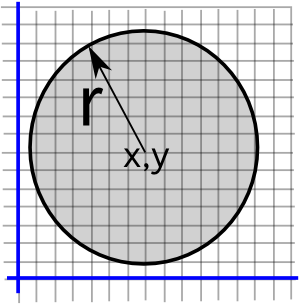(x - x1)² + (y - y1)² < r² |z-d| < r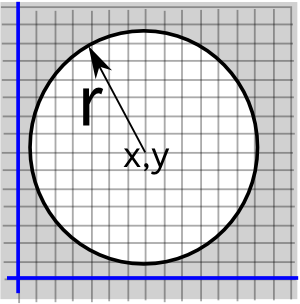(x - x1)² + (y - y1)² > r² |z-d| > rtan-1(y/x) = θ arg(z)=θ

z plane   w plane-->

w=z²-->

w=ez-->

w=1/zSpecific information about these functions is shown in the complex number part of the site (functions of a complex variable).

### Cauchy's Theorem

If f(z) is analytic in a region R (no poles in R) then: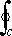f(z) dz = 0

### Extension of Cauchys Theorem

∫ round same singularities are equal.

∫ round several singularities = sum of ∫ round each singularity.

### Use of Cauchys Theorem to evaluate Unknown Integral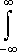e-x² = √πe-x² cos(2 b x) dx = √π ee-x² sin(2 b x) dx = 0

proof that:e-z² dz - > 0 as a - > ∞

principles used:

1. |f(z) dz | <=| f(z) dz |
2.|f(z) dz | =| f(z)| |dz | <= ml

## Poles of a function

A function can have several laurent series each corresponding to a pole:

Laurent Series:

f(z) = an/(z-a)n + an-1/(z-a)n-1 + … +a1/(z - a) + b0 + b1(z-a) + …

where:

• a = a pole of function
• a1 = residue of pole

### Evaluation of residue at simple pole

1. multiply by (z-pole)
2. take limit as z -> a

i.e. a1 = limz->a(z - a) f(z)

### Evaluation of residue at pole of order n

a1 = 1/(n-1)! limz->a dn-1/ d zn-1{(z - a)n f(z)}

### Application of poles: complex integrationf(z) d z = 2 π i (sum of enclosed resides)

### Evaluation of integrals between ±∞

1. Find Poles
2. Take suitable path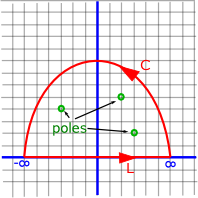3. Find residues of poles inside path
4. Check if=0 (denominator of f(z) 2 more than numerator) (proof uses≤ml)

Where I can, I have put links to Amazon for books that are relevant to the subject, click on the appropriate country flag to get more details of the book or to buy it from them.Visual Complex Analysis - If you already know the basics of complex numbers but want to get an in depth understanding using an geometric and intuitive approach then this is a very good book. The book explains how to represent complex transformations such as the Möbius transformations. It also shows how complex functions can be differentiated and integrated.